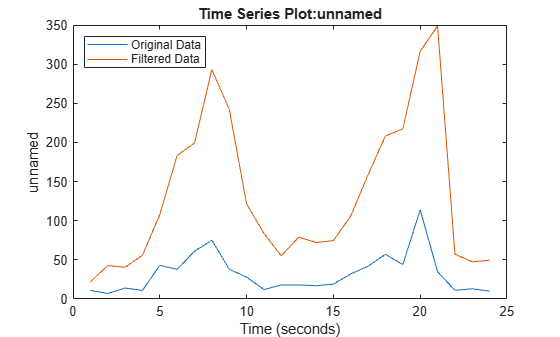Documentation

# filter

Modify frequency content of `timeseries` objects

## Syntax

``tsout = filter(tsin,b,a)``
``tsout = filter(tsin,b,a,ind)``

## Description

````tsout = filter(tsin,b,a)` applies the rational transfer function filter b(z−1)/a(z−1) to the uniformly-spaced data in the `timeseries` object `tsin`. The numerator `b` and denominator `a` are vectors containing the transfer function coefficients.```
````tsout = filter(tsin,b,a,ind)` specifies the indices of the columns or rows to filter. `ind` is a vector of integers representing column indices for column-oriented data (`tsin.IsTimeFirst` is `true`) and representing row indices for row-oriented data (`tsin.IsTimeFirst` is `false`).```

## Examples

collapse all

This example applies the following transfer function to a set of data:

`$H\left({z}^{-1}\right)=\frac{b\left({z}^{-1}\right)}{a\left({z}^{-1}\right)}=\frac{2+3{z}^{-1}}{1+0.2{z}^{-1}}$`

Create a `timeseries` object from the matrix `count` in `count.dat`.

```load count.dat tsin = timeseries(count(:,1),[1:24]);```

Enter the coefficients for the denominator and numerator of the transfer function. Order the coefficients in ascending powers of ${z}^{-1}$ to represent $1+0.2x$ and $2-3{z}^{-1}$.

```a = [1 0.2]; b = [2 3];```

Apply the transfer function using `filter`, and compare the original data to the filtered data.

```tsout = filter(tsin,b,a); plot(tsin) hold on plot(tsout) legend('Original Data','Filtered Data','Location','NorthWest')```## Input Arguments

collapse all

Input `timeseries`, specified as a scalar. `tsin` must be uniformly sampled.

Data Types: `timeseries`

Numerator coefficients of the transfer function, specified as a scalar or vector.

Denominator coefficients of the transfer function, specified as a scalar or vector.

Row or column indices, specified as a positive integer numeric scalar or vector. `ind` represents column indices for column-oriented data (`tsin.IsTimeFirst` is `true`) and represents row indices for row-oriented data (`tsin.IsTimeFirst` is `false`).

Data Types: `double` | `single` | `int8` | `int16` | `int32` | `int64` | `uint8` | `uint16` | `uint32` | `uint64`

collapse all

### Rational Transfer Function

The input-output description of the `filter` operation on a vector in the Z-transform domain is a rational transfer function. A rational transfer function is of the form,

`$Y\left(z\right)=\frac{b\left(1\right)+b\left(2\right){z}^{-1}+...+b\left({n}_{b}+1\right){z}^{-{n}_{b}}}{1+a\left(2\right){z}^{-1}+...+a\left({n}_{a}+1\right){z}^{-{n}_{a}}}X\left(z\right),$`

which handles both FIR and IIR filters . na is the feedback filter order, and nb is the feedforward filter order.

You also can express the rational transfer function as the following difference equation,

`$\begin{array}{c}a\left(1\right)y\left(n\right)=b\left(1\right)x\left(n\right)+b\left(2\right)x\left(n-1\right)+...+b\left({n}_{b}+1\right)x\left(n-{n}_{b}\right)\\ -a\left(2\right)y\left(n-1\right)-...-a\left({n}_{a}+1\right)y\left(n-{n}_{a}\right).\end{array}$`

Furthermore, you can represent the rational transfer function using its direct form II transposed implementation, as in the following diagram. Due to normalization, assume `a(1) = 1`.The operation of `filter` at sample m is given by the time domain difference equations